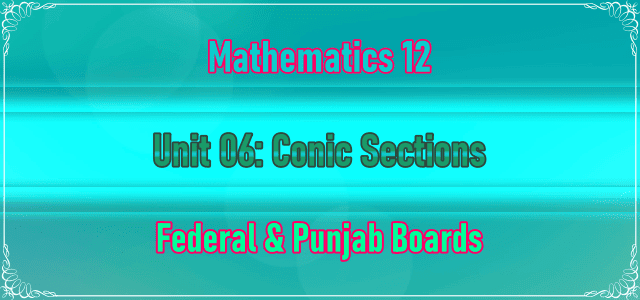# 2nd Year Math Chapter 6 Notes | Unit 06: Conic Section2nd Year Math Chapter 6 Notes
2nd Year Math Chapter 6 Notes | Unit 06: Conic Section are being uploaded here. These F.Sc. Math part 2 chapter no. 6: Conic Section notes are in accordance with the syllabus and paper pattern of Punjab Text Book Board Lahore. These notes are very useful for the students belong to the Sargodha board, Lahore Board, Sahiwal Board, Multan Board, Kashmir board, Rawalpindi board, Gujranwala board, and DG Khan board.

### 2nd Year Math Chapter 6 Notes | Unit 06: Conic Section

You can easily view or download these notes. If you want to view 12th class Math chapter 6 notes online, there must be a PDF reader installed on your computer. These notes cover the following topics
• Introduction
• Equation of a Circle
• General form of an Equation of a Circle
• Equation of a Circle Determined by given Conditions
• Exercise 6.1
• Tangents and Normals
• Length of the Tangent to a Circle (Tangential distance)
• Analytic Proofs of Important Properties of a Circle
• Parabola
• General form of an Equation of a Parabola
• Other Standard Parabolas
• Graph the Parabola
• Ellipse and its Elements
• Standard form of an Ellipse
• Graph of an Ellipse
• Hyperbola and its Elements
• Standard Equation of Hyperbola
• Graph of the Hyperbola
• Tangents and Normals
• Intersection of two Conics
• Translation and Rotation of Axes
To view online or download 2nd year Math chapter 6 notes in PDF Format, click the button given below. Don't forget to share these notes with your friend, class fellows, and teachers.